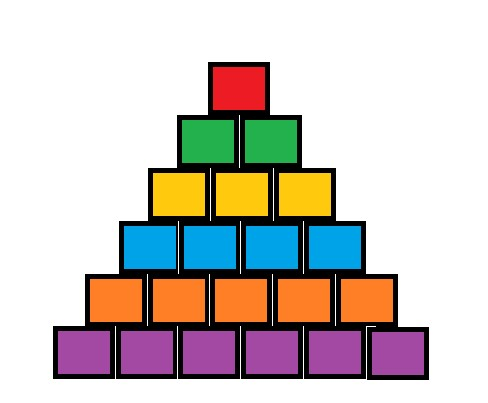시간 제한 메모리 제한 제출 정답 맞은 사람 정답 비율
1 초 512 MB 1 1 1 100.000%

## 문제Suppose that you are an admiral of a famous naval troop. The troop consists of 21 battleships. There are 6 kinds of battleships. First, there is one flagship in which the admiral must be, and it is denoted by the integer 0. Other battleship kinds are denoted by integers from 1 to 5, and there are 2, 3, 4, 5, and 6 ships of these kinds, respectively.

The troop is going to a battle against the enemy. Hence, the correct arrangement of the battleships is very important. The shape of the battlefield is shown on the picture to the right. For simplicity, we consider all battleships to have the same rectangular shape.

Fortunately, the optimal arrangement of the battleships is already known. As you can see, the battlefield consists of 6 rows. In the optimal arrangement, all the battleships denoted by number $i$ must be located in the $i$-th row (rows are numbered starting from $0$).

You are given the initial state of the battlefield as input. The battleships occupy the 21 required positions, but some of them may be in a wrong row. You can change the state of battlefield by swapping the position of the flagship with an adjacent battleship. Two battleships are considered adjacent if and only if they are not in the same row, but share parts of their edges. For example, if we denote a cell in $i$-th row and $j$-th position from the left as $(i, j)$, then the cell $(2, 1)$ is adjacent to the cells $(1, 0)$, $(1, 1)$, $(3, 1)$, and $(3, 2)$ (here, rows and positions are numbered starting from $0$). Your task is to make the minimum possible number of swaps so as to reach the optimal arrangement, or to report that more than $20$ swaps are required.

## 입력

The first line of input contains an integer $T$, the number of test cases ($1 \le T \le 10$).

Each test case consists of 6 lines. The $i$-th line of each test case contains $i$ space-separated integers denoting the kinds of battleships at the $i$-th row of the battlefield, listed from left to right.

## 출력

For each test case, if you can't reach the goal in 20 or less swaps, print "too difficult" on a single line. Otherwise, print one integer: the minimum possible number of swaps.

## 예제 입력 1

1
1
2 0
2 1 2
3 3 3 3
4 4 4 4 4
5 5 5 5 5 5


## 예제 출력 1

3